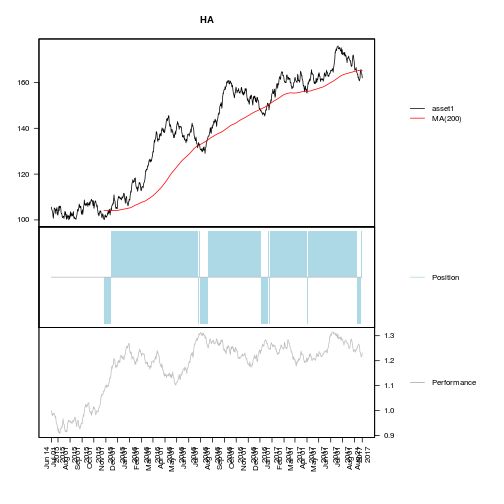``````library(xts)
``````
``````## Loading required package: zoo
``````
``````##
## Attaching package: 'zoo'
``````
``````## The following objects are masked from 'package:base':
##
##     as.Date, as.Date.numeric
``````

## Introduction

This package allows users to apply customizable quantitative trading strategies to historical portfolio data. An S4 class called “Strategy” is implemented that creates objects from which vaious performance measurements may be extracted, visualized and compared. A template for custom quantitative strategies is available and so the package can be extended in an easy way and still all methods are available for consistent evaluations.

## Structure of `Strategy`-Objects

A `Strategy`-object contains all relevant information about the strategy executed on the portfolio data. Dependent packages are `zoo` and `xts`.

## Data

There is no data available within this package.

## Examples

``````library(Strategy)

# Generate positive random walks for random assets
set.seed(2)
len <- 1000
n <- 10
assets <- abs(apply(matrix(rnorm(n*len), ncol=n), 2, cumsum)) + 100
colnames(assets) <- paste0("asset", 1:n)
assets <- xts(assets, order.by = seq(from=Sys.Date()-len, length.out=len, by="d"))

# MA(200)-strategy
myStrat.MA200 <- Strategy(assets = assets
, strat = "MA"
, strat.params = list(k=200))
``````“He who gives up [code] safety for [code] speed deserves neither.” (via)